• 1 逻辑回归多分类我们已经知道，普通的logistic回归只能针对二分类(Binary Classification)问题，要想实现多个类别的分类，我们必须要改进logistic回归，让其适应多分类问题。关于这种改进，有两种方式可以做到。...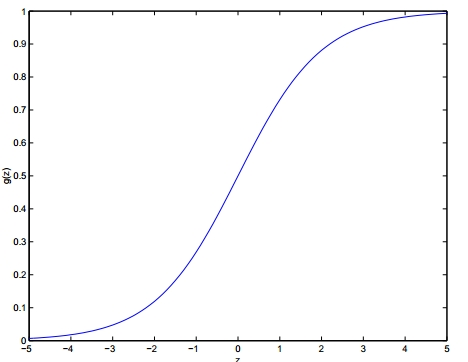1 逻辑回归与多分类我们已经知道，普通的logistic回归只能针对二分类(Binary Classification)问题，要想实现多个类别的分类，我们必须要改进logistic回归，让其适应多分类问题。关于这种改进，有两种方式可以做到。第一种方式是直接根据每个类别，都建立一个二分类器，带有这个类别的样本标记为1，带有其他类别的样本标记为0。假如我们有k个类别，最后我们就得到了k个针对不同标记的普通的logistic二分类器。（本质上就是ovr的做法）第二种方式是修改logistic回归的损失函数，让其适应多分类问题。这个损失函数不再笼统地只考虑二分类非1就0的损失，而是具体考虑每个样本标记的损失。这种方法叫做softmax回归，即logistic回归的多分类版本。2 ovr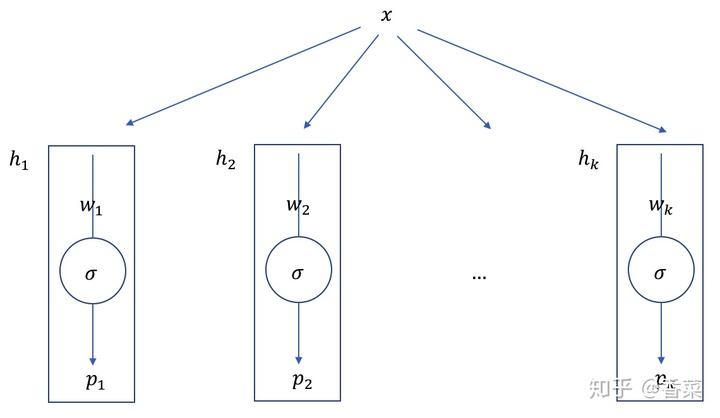对于二分类问题，我们只需要一个分类器即可，但是对于多分类问题，我们需要多个分类器，其中表示一个二分类模型，其判断样本属于第类的概率值。对于的训练，我们挑选出带有标记为的样本标记为1，将剩下的不带标记的样本标记为0。针对每个分类器，都按上述步骤构造训练集进行训练。针对每一个测试样本，我们需要找到这k个分类函数输出值最大的那一个，即为测试样本的标记3 softmax该模型将逻辑回归推广到分类问题，其中类标签y可以采用两个以上的可能值。这对于诸如MNIST数字分类之类的问题将是有用的，其中目标是区分10个不同的数字。Softmax回归是一种监督学习算法，但我们稍后会将其与我们的深度学习/无监督特征学习方法结合使用。在softmax回归设置中，我们对多类分类感兴趣（而不是仅对二元分类），所以y可以取k个不同的取值。因此，在我们的训练集，其中。给定测试输入x，我们希望我们的模型估计每个类别的概率。因此，我们的模型将输出k维向量（其元素总和为1），给出我们的k个类别的估计概率。具体地说，我们的假设$h_theta(x)$采用以下形式：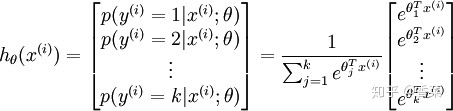其中，是模型的参数，而是归一化项。为方便起见，我们还会向量法来表示模型的所有参数。当你实现softmax回归时，将θ表示为通过堆叠成行获得的k-by（n + 1）矩阵通常很方便，这样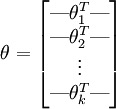损失函数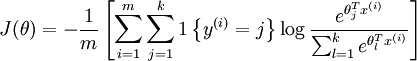求导后，可得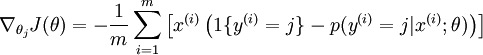更新参数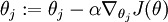4 ovr vs. softmax假设您正在处理音乐分类应用程序，并且您正在尝试识别k种类型的音乐。您应该使用softmax分类器，还是应该使用逻辑回归构建k个单独的二元分类器？这取决于这四个类是否相互排斥。例如，如果您的四个类是经典，乡村，摇滚和爵士乐，那么假设您的每个训练样例都标有这四个类别标签中的一个，那么您应该构建一个k = 4的softmax分类器。（如果有'还有一些不属于上述四个类的例子，那么你可以在softmax回归中设置k = 5，并且还有第五个，“以上都不是”类。）但是，如果你的类别是has_vocals，舞蹈，配乐，流行音乐，那么这些课程并不相互排斥;例如，可以有一段来自音轨的流行音乐，另外还有人声。在这种情况下，构建4个二元逻辑回归分类器更合适。这样，对于每个新的音乐作品，您的算法可以单独决定它是否属于四个类别中的每一个。现在，考虑一个计算机视觉示例，您尝试将图像分为三个不同的类。（i）假设您的课程是indoor_scene，outdoor_urban_scene和outdoor_wilderness_scene。你会使用sofmax回归还是三个逻辑回归分类器？（ii）现在假设你的课程是indoor_scene，black_and_white_image和image_has_people。您会使用softmax回归或多重逻辑回归分类器吗？在第一种情况下，类是互斥的，因此softmax回归分类器是合适的。在第二种情况下，构建三个单独的逻辑回归分类器更为合适。总结就是，如果类别之间是互斥的，那么用softmax会比较合适，如果类别之间不是互斥的，用ovr比较合适。
展开全文• matlab实现的多分类逻辑回归，用于手写数字识别
• matlab开发-分类逻辑回归。二类和分类的Logistic回归
• LR逻辑回归算法应用于多分类逻辑回归LR算法介绍逻辑回归LR的多分类方法方法1：1对多方法2：1对1方法3：softmax逻辑回归LR的matlab程序 逻辑回归LR算法介绍 逻辑回归算法，常用于二分类{0，1}，matlab的有相应的函数...
LR逻辑回归算法应用于多分类逻辑回归LR算法介绍逻辑回归LR的多分类方法方法1：1对多方法2：1对1方法3：softmaxLR逻辑回归的matlab程序
逻辑回归LR算法介绍
逻辑回归算法，常用于二分类{0，1}，matlab的有相应的函数可以求得二分类的系数，怎么才能用于多分类？
逻辑回归LR的多分类方法
方法1：1对多
1.训练时：依次将其中的一类看作正例，将其他的所有类看成负例，进行二分类；若有$n$个类别，则需要有$n$个二分类器；
2.测试时：将测试数据分别导入所有的二分类器中，对所有的类别进行投票，若测试数据被分为某类，则该类投票数加1，投票数最多的为判别类别；
方法2：1对1
1.训练时：两两类别间建立二分类器，将其中一类看成正例，另一类看成反例；若有$n$个类别，则需要有$\frac {n(n-1)}{2}$个；
2.测试时：将样本输入到每个二分类器进行运算，也是按照投票的方式进行选择，投票数最大的为判别类别；
方法3：softmax
采用softmax函数，直接可以映射到多个0到1之间，是二分类的扩展；
LR逻辑回归的matlab程序
b =glmfit(train_data,[train_label,ones(9,1)],'binomial', 'link', 'logit');
p = glmval(b,train_data, 'logit');%对所有的样本进行测试


引用：参考链接


展开全文机器学习
• 概念笔记：多分类逻辑回归代码实现：""" 多类分类 使用逻辑回归来识别手写数字0-9 """ import numpy as np import pandas as pd import matplotlib.pyplot as plt from scipy.io import loadmat # 用于读取matlab...
概念笔记：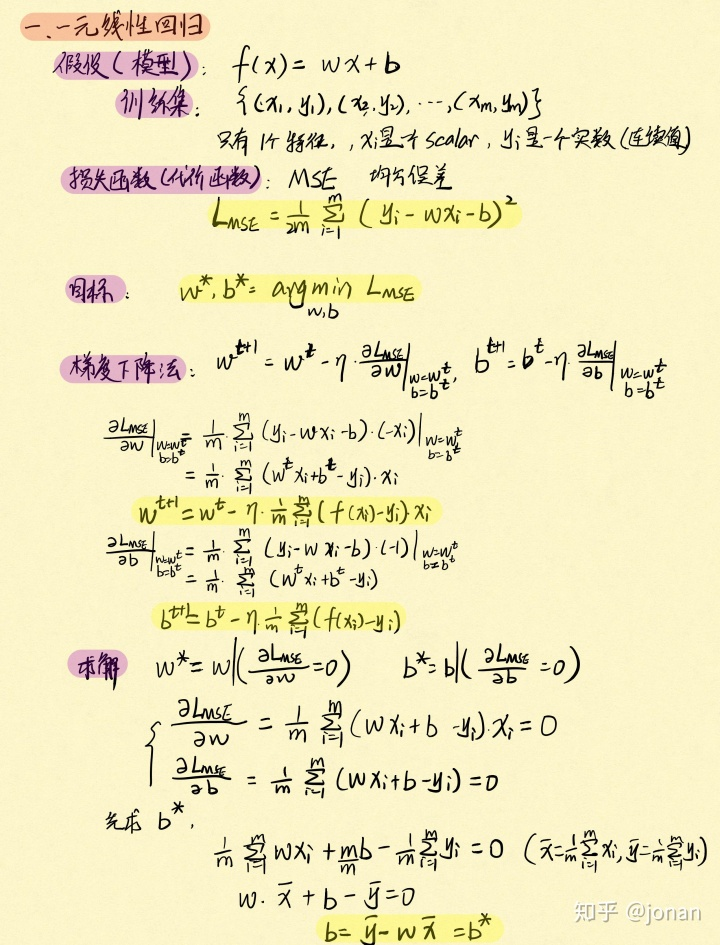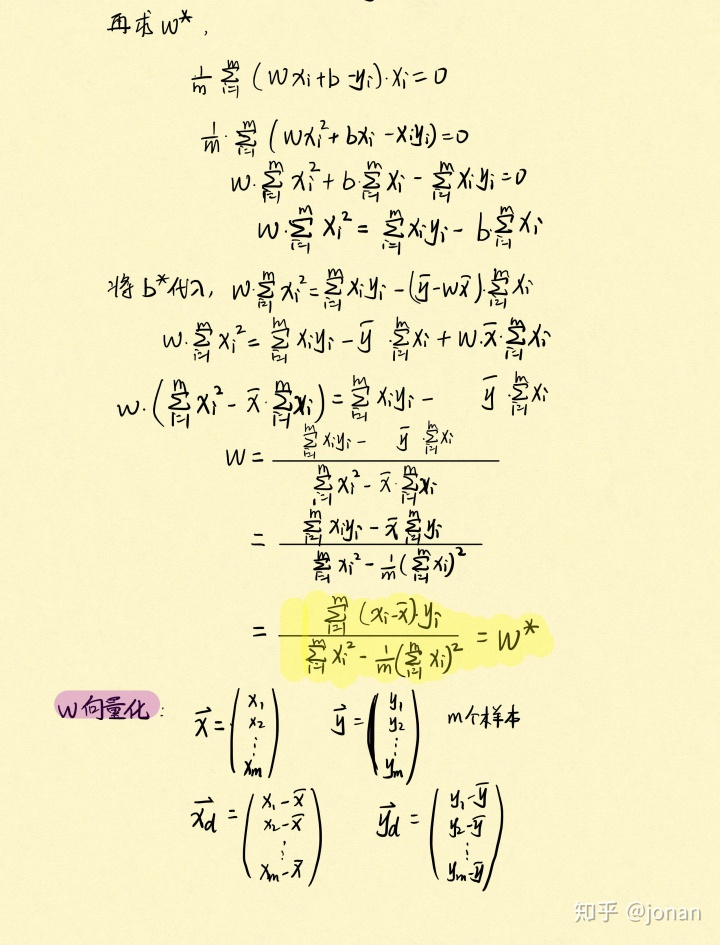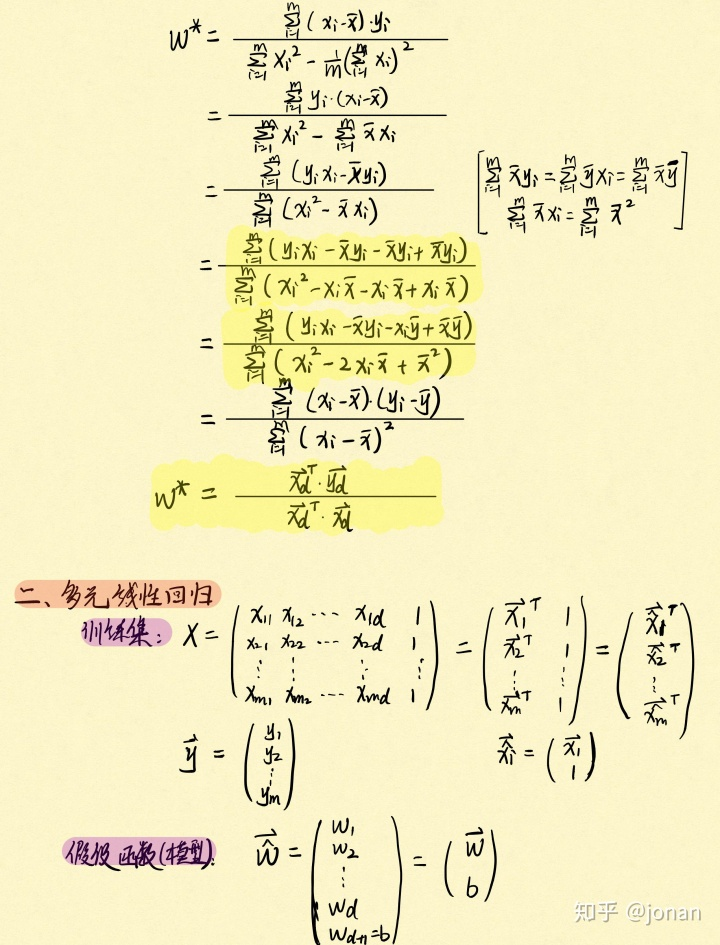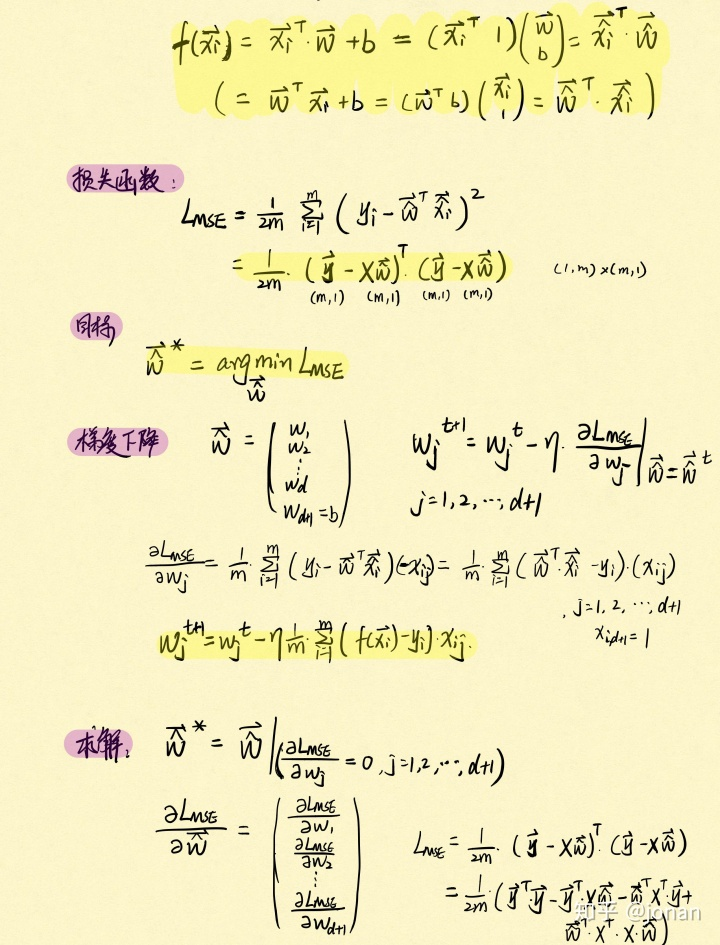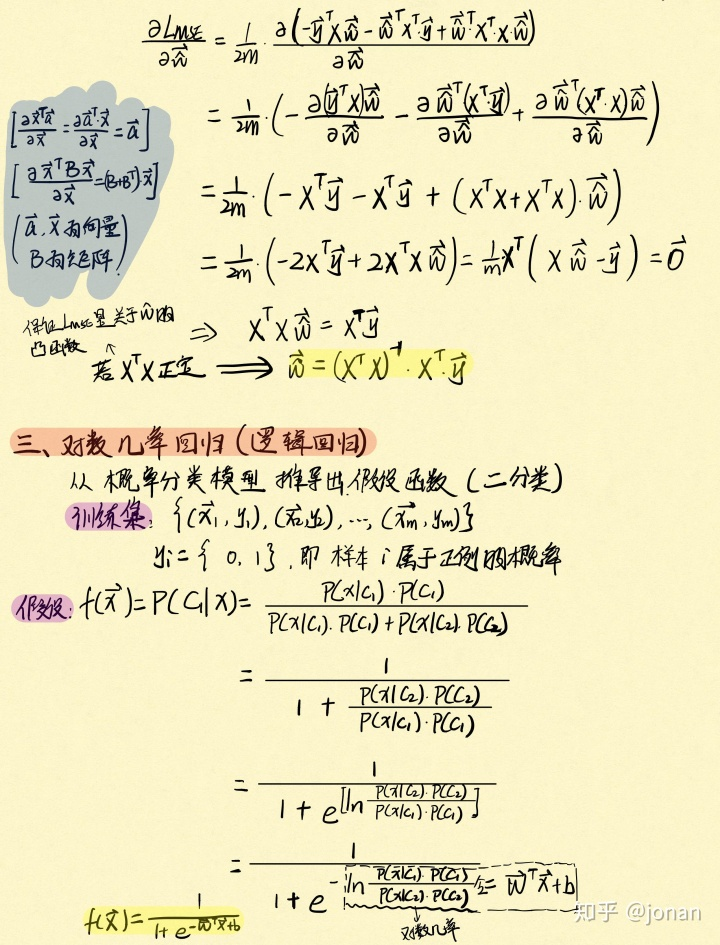多分类逻辑回归代码实现："""
多类分类
使用逻辑回归来识别手写数字0-9
"""

import numpy as np
import pandas as pd
import matplotlib.pyplot as plt
from scipy.io import loadmat  # 用于读取matlab数据文件

# 准备数据
data = loadmat('ex3data1.mat')

# print(data)
# print(data['X'].shape, data['y'].shape)  # (5000, 400) (5000, 1)

# 第一个任务：将逻辑回归实现完全向量化

# sigmoid函数
def sigmoid(z):
return 1 / (1 + np.exp(-z))

# 代价函数
def cost(theta, X, y, learningRate):
"""
:param theta: 参数, shape [1 + feature_num, ]
:param X: 输入特征, shape [sample_num, 1 + feature_num]
:param y: 输出类别, shape [sample_num, ]
:param learningRate: 正则化参数, scalar
:return: whole_cost: 损失值, scalar
"""
# 将theta、X、y转为numpy矩阵
theta = np.array(theta)  # shape [1 + feature_num, ]
X = np.array(X)  # shape [sample_num, 1 + feature_num]
y = np.array(y)  # shape [sample_num, ]

# 根据公式计算损失函数（不含正则化）
predict = sigmoid(X @ theta.T)  # shape [sample_num, ]
cross_cost = -y * np.log(predict) - (1 - y) * np.log(1 - predict)
# cross_cost: shape [sample_num, ]

# 根据公式计算损失函数中的正则化部分
reg = (learningRate / (2 * len(X))) * np.sum(np.power(theta[1:], 2))  # scalar

# 将上述结果相加
whole_cost = np.sum(cross_cost) / len(X) + reg  # scalar

return whole_cost

# theta = np.zeros([1 + 400, ])
# X = np.insert(data['X'], 0, values=np.ones([5000, ]), axis=1)
# y_0 = np.array([1 if label == 1 else 0 for label in data['y'].ravel()]).reshape([len(X), ])
# print(cost(theta, X, y_0, 1))

# 向量化的梯度下降法
def gradient(theta, X, y, learningRate):
"""
:param theta: 参数, shape [1 + feature_num, ]
:param X: 输入特征, shape [sample_num, 1 + feature_num]
:param y: 输出类别, shape [sample_num, ]
:param learningRate: 学习率, scalar
:return: dtheta: 梯度，shape [1 + feature_num, ]
"""
# 将theta、X、y转为numpy矩阵
theta = np.array(theta)  # shape [1 + feature_num, ]
X = np.array(X)  # shape [sample_num, 1 + feature_num]
y = np.array(y)  # shape [sample_num, ]

# 获得要更新的参数量
parameters = int(theta.ravel().shape)  # ravel降维打击，将所有元素降到一维

# 计算预测的误差
predict = sigmoid(np.dot(X, theta.T))  # shape [sample_num, ]
error = predict - y  # shape [sample_num, ]

# 根据error计算梯度
dtheta = np.dot(error, X).reshape(theta.shape) / len(X) + (learningRate / len(X)) * theta
# dtheta: shape [1 + feature_num, ]

# 由于b不需要正则化，需要重置b的梯度
dtheta -= (learningRate / len(X)) * theta

return dtheta  # shape [1 + feature_num, ]

# 基于上面定义的代价函数和梯度函数，接下来构建模型训练的函数。
# 该任务有10个可能的类，并且逻辑回归一次只能在2类间进行分类，
# 采用的解决办法是训练10个分类器，每个分类器在“是类别i”和“不是类别i”之间进行分类，i=1...10
# 把10个分类器的训练过程集成在一个函数中，该函数计算10个分类器最终权重，返回为10*(1+feature_num)的numpy数组
from scipy.optimize import minimize

def one_vs_all(X, y, num_labels, learning_rate):
"""
:param X: 输入特征, shape [sample_num, feature_num]
:param y: 输出类别, shape [sample_num, ]
:param num_labels: 标签数, scalar
:param learning_rate: 学习率, scalar
:return: all_theta, shape [num_labels, 1 + feature_num]
"""
rows = X.shape  # sample_num
params = X.shape  # feature_num

# 初始化权重参数为0
all_theta = np.zeros([num_labels, 1 + params])

# 在X的最左边插入一列1，表示x0
X = np.insert(X, 0, values=np.ones([rows, ]), axis=1)  # shape [sample_num, 1 + feature_num]

# 训练阶段，训练10个分类器
for i in range(1, num_labels + 1):
theta = np.zeros([1 + params, ])  # shape [1 + feature_num, ]
y_i = np.array([1 if label == i else 0 for label in y]).reshape([rows, ])  # shape [sample_num, ]

# 最小化目标函数（代价函数）
# X:shape [sample_num, 1 + feature_num], y_i:shape [sample_num, ]
fmin = minimize(fun=cost, x0=theta, args=(X, y_i, learning_rate), method='TNC', jac=gradient)
# scipy.minimize会自动把theta转为[1+feature_num,]的shape，所以gradient的输出也需要是[1+feature_num,]
all_theta[i - 1, :] = fmin.x

return all_theta  # shape [num_labels, 1 + feature_num]

all_theta = one_vs_all(X=data['X'], y=data['y'], num_labels=10, learning_rate=1)

# print(all_theta)

# 使用训练完毕的分类器预测每个图像的标签。需要计算每个类的类概率，输出对应样本的最高概率的类标签
def predict_all(X, all_theta):
"""
:param X: 输入, shape: [sample_num, feature_num]
:param all_theta: 10个分类器的参数矩阵, shape [10, 1 + feature_num]
:return: h_argmax: 所有样本预测结果, shape [sample_num, 1]
"""
# 获取输入矩阵的维度信息
rows = X.shape  # sample_num
params = X.shape  # feature_num
num_labels = all_theta.shape  # num_labels

# 把矩阵X加入一列0元素
# X = np.insert(X, , values=np.ones([rows, 1]), axis=1)
X = np.insert(X, 0, values=np.ones([rows, ]), axis=1)  # shape [sample_num, 1 + feature_num]

# 把矩阵X和all_theta转换为numpy矩阵
X = np.array(X).reshape([rows, 1 + params])  # shape [sample_num, 1 + feature_num]
all_theta = np.array(all_theta).reshape([num_labels, 1 + params])  # shape [num_labels, 1 + feature_num]

# 计算样本属于每一类的概率
h = sigmoid(np.dot(X, all_theta.T))  # shape [sample_num, num_labels]

# 找到每个样本中预测概率最大的值对应的索引
h_argmax = np.argmax(h, axis=1)  # shape [sample_num, 1]

# 索引+1才是对应的预测标签
h_argmax = h_argmax + 1  # shape [sample_num, 1]

return h_argmax  # shape [sample_num, 1]

y_pred = predict_all(data['X'], all_theta)  # shape [sample_num, ]
correct = [1 if a == b else 0 for (a, b) in zip(y_pred, data['y'])]  # shape [sample_num, ]
accuracy = (sum(map(int, correct))) / float(len(correct))
print('accuracy = {}%'.format(accuracy * 100))
展开全文• 作业任务项一：多分类逻辑回归 代码如下： load('E:\研究生\机器学习\吴恩达机器学习python作业代码\code\ex3-neural network\ex3data1.mat'); m=size(X,1); rand_idices=randperm(m); sel=X(rand_idices(1:100),:);...
作业任务项一：多分类逻辑回归
代码如下：
load('E:\研究生\机器学习\吴恩达机器学习python作业代码\code\ex3-neural network\ex3data1.mat');
m=size(X,1);
rand_idices=randperm(m);
sel=X(rand_idices(1:100),:);
displayData(sel);

randperm是把m个自然数打乱的函数，sel从X中抽出100个被打乱的实例进行展示。
其中displayData.m如下：
function [h, display_array] = displayData(X, example_width)
%DISPLAYDATA Display 2D data in a nice grid
%   [h, display_array] = DISPLAYDATA(X, example_width) displays 2D data
%   stored in X in a nice grid. It returns the figure handle h and the
%   displayed array if requested.

% Set example_width automatically if not passed in
if ~exist('example_width', 'var') || isempty(example_width)
example_width = round(sqrt(size(X, 2)));
end

% Gray Image
colormap(gray);

% Compute rows, cols
[m n] = size(X);
example_height = (n / example_width);

% Compute number of items to display
display_rows = floor(sqrt(m));
display_cols = ceil(m / display_rows);

% Between images padding
pad = 1;

% Setup blank display
display_array = - ones(pad + display_rows * (example_height + pad), ...
pad + display_cols * (example_width + pad));

% Copy each example into a patch on the display array
curr_ex = 1;
for j = 1:display_rows
for i = 1:display_cols
if curr_ex > m
break;
end
% Copy the patch

% Get the max value of the patch
max_val = max(abs(X(curr_ex, :)));
display_array(pad + (j - 1) * (example_height + pad) + (1:example_height), ...
pad + (i - 1) * (example_width + pad) + (1:example_width)) = ...
reshape(X(curr_ex, :), example_height, example_width) / max_val;
%(2,2)~(21,21)录入20*20的矩阵,(2,23)~(2,42)录入20*20的矩阵，以此类推
curr_ex = curr_ex + 1;
end
if curr_ex > m
break;
end
end

% Display Image
h = imagesc(display_array, [-1 1]);

% Do not show axis
axis image off

drawnow;

end


其中if语句是如果没有填写单个图像宽度或者是填写的宽度不是向量形式，则使单个图像宽度为X的列数开方并圆整。实际上X是单个图像像素矩阵展成的向量形式，例如一个图像像素矩阵是20*20，那么展成向量形式为1*400，因此该步骤实际上是在还原单个像素矩阵。
colormap是让整个矩阵的色调变为灰色。从-1到1之间变化，-1为纯黑色，1为纯白色。
紧接着是计算单个像素矩阵的高度，总矩阵的宽度和高度。
floor为向下取整（地板），如5.1和5.9都取5 。
ceil为向上取整（天花板），如5.1和5.9都取6 。
round是四舍五入，如5.1取5，5.9取6 。
此时如果单个像素矩阵为20*20，一共展示100个图像，那么理论上总矩阵应该是200*200，但是由于展示的图像都是灰底，因此要加上边框。因此总矩阵应该为211*211。程序中的做法为：先创建一个纯黑的矩阵，然后再往其中填单个图像矩阵。其中pad + (j - 1)(example_height + pad) + (1:example_height)的意义是：pad是边，中间的(j - 1)(example_height + pad)是已经填过的图像的高度，(1:example_height)是现在要填的图像的高度。
接下来是多分类逻辑回归部分：
首先是测试部分，验证IrCostFunction.m正确与否。验证正确后，应将验证部分注释。
theta_t = [-2; -1; 1; 2];
X_t = [ones(5,1) reshape(1:15,5,3)/10];
y_t = ([1;0;1;0;1] >= 0.5);
lambda_t = 3;
[J,grad] = IrCostFunction(X_t, y_t,theta_t, lambda_t);
fprintf('\nCost: %f\n', J);
fprintf('Expected cost: 2.534819\n');
fprintf('Gradients:\n');
fprintf(' %f \n', grad);
fprintf('Expected gradients:\n');
fprintf(' 0.146561\n -0.548558\n 0.724722\n 1.398003\n');

其中IrCostFunction.m如下：
公式如下：$J(\theta)=-\frac{1}{m}\sum_{i=1}^m[y^{(i)}log(h_\theta(x^{(i)}))+(1-y^{(i)})log(1-h_\theta(x^{(i)}))]+\frac{\lambda}{2m}\sum_{j=1}^n\theta_j^2$
function [J,grad]=IrCostFunction(X,y,theta,lambda)
J=0;
m=length(y);
grad=zeros(size(theta));
theta_1=[0;theta(2:end,1)];
J=1/m*(-y'*log(sigmoid(X*theta))-(1-y)'*log(1-sigmoid(X*theta)))+lambda/(2*m)*(theta_1'*theta_1);
grad=1/m*X'*(sigmoid(X*theta)-y)+lambda/m*theta_1;
end

其中sigmoid.m如下：
function g=sigmoid(z)
g=zeros(size(z));
g=1./(1+exp(-z));
end

多分类处理部分如下：
num_labels是图像的数，其中0被10代替。经过初始化后，进行多分类处理。
num_labels=10;
lambda=0.1;
[All_theta]=oneVsAll(X,y,num_labels,lambda);
p=PredictOneVsAll(All_theta,X);
fprintf('\nTraining Set Accuracy: %f\n', mean(double(p == y)) * 100);


得到p为预测的数字向量，与标签进行比对，可以获得最终正确率为95.020000。
其中oneVsAll.m如下：
function [All_theta]=oneVsAll(x,y,num_labels,lambda)
m=size(x,1);
X=[ones(m,1),x];
All_theta=zeros(num_labels,size(X,2));
initial_theta=zeros(size(X,2),1);
option=optimset('Gradobj','on','MaxIter',50);
for c=1:num_labels
[theta]=fmincg(@(t)IrCostFunction(X,(y==c),t,lambda),initial_theta,option);
All_theta(c,:)=theta';
end


由于多分类等于有多个二分类器，因此这里的All_theta储存了10个二分类器的theta变量，其中的theta是转置放置的。fmincg是一个高级优化算法，其代码可以在网上找到。其中(y==c)是指当样本标签和预设的c相同的时候置1 。示意如表所示：

1
→
1
0
…
0

2
→
0
1
…
0

3
→
0
0
…
0

…
…
…
…
…
…

9
→
0
0
…
0

10
→
0
0
…
1

其中PredictOneVsAll.m如下：
function p=PredictOneVsAll(All_theta,x)
m=size(x,1);
X=[ones(m,1),x];
p=zeros(size(X,1),1);
temp=zeros(size(X,1),size(All_theta,1));
for i=1:size(All_theta,1)
temp(:,i)=sigmoid(X*(All_theta(i,:))');
end
for i=1:size(X,1)
[~,ix]=max(temp(i,:));
p(i)=ix;
end


其中temp中的每一行为一个实例，列为十个预测1~10，其中的每个数为该预测的假设函数值。再选择最大的假设函数值所对应的列标签，可以得到p为该实例最后预测的数字。
作业任务项二：神经网络
代码如下：
load('E:\研究生\机器学习\吴恩达机器学习python作业代码\code\ex3-neural network\ex3weights.mat')
load('E:\研究生\机器学习\吴恩达机器学习python作业代码\code\ex3-neural network\ex3data1.mat')
% Theta1 has size 25 x 401
% Theta2 has size 10 x 26
p=predict(Theta1,Theta2,X);
fprintf('\nTraining Set Accuracy: %f\n', mean(double(p == y)) * 100);

由于Theta1和Theta2都是预设好的，因此无需进行训练模型的操作。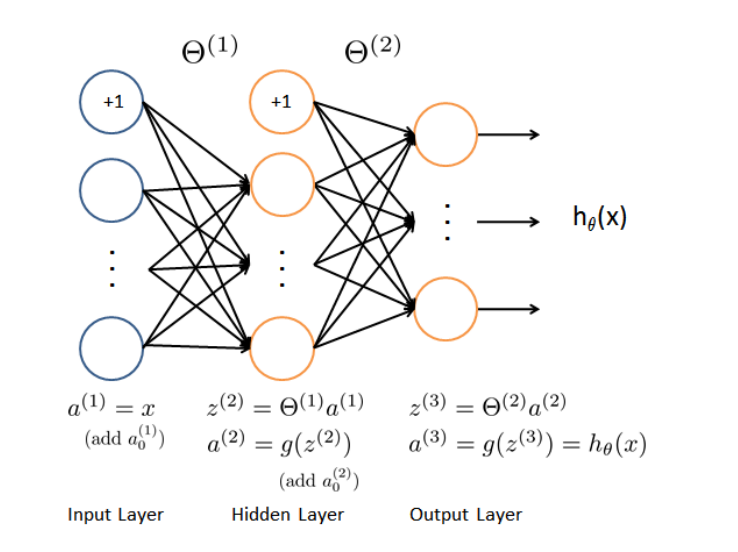其中predict.m如下：
function p=predict(Theta1,Theta2,x)
X=[ones(size(x,1),1),x];
m=size(X,1);
p=zeros(size(X,1),1);
z_2=X*Theta1';
a_2=sigmoid(z_2);
a_2=[ones(size(a_2,1),1),a_2];
z_3=a_2*Theta2';
a_3=sigmoid(z_3);
[~,p]=max(a_3,[],2);

最终可以获得正确率为97.520000。


展开全文机器学习 神经网络
• 我们将扩展我们在练习2中写的逻辑回归的实现，并将其应用于一对一的分类。 让我们开始加载数据集。 它是在MATLAB的本机格式，所以要加载它在Python，我们需要使用一个SciPy工具。 import numpy as np import pandas...python 机器学习
• 逻辑回归多分类问题——识别手写数字了解.mat文件读取数据划分集合测试图片 了解.mat文件 .mat文件是属于matlab的文件，具体有什么特点和属性还不知道，但对于本题中我们需要去读取，要用到python中的Scipy库下的...
• 二元逻辑回归介绍定义Logistic回归主要用于因变量为分类变量（如是否等）的回归分析，自变量可以为分类变量，也可以为连续变量。它可以从个自变量中选出对因变量有影响的自变量，并可以给出预测公式用于预测。而因...
• 下面使用逻辑回归实现多分类问题：识别手写的阿拉伯数字(0-9)   数据加载到Matlab中的格式如下：   一共有5000个训练样本，每个训练样本是400维的列向量（20X20像素的 grayscale i
• 多类别逻辑回归多分类数据集将逻辑回归向量化sigmoid 函数 代价函数向量化正则化逻辑回归一对多分类器一对多预测 多分类 多分类 这个部分需要你实现手写数字（0到9）的识别。你需要扩展之前的逻辑回归，并将其应用...python 机器学习 神经网络
• 1 多分类 这个部分需要你实现手写数字（0到9）的识别。...你将用多分类逻辑回归做一个分类器。因为现在有10个数字类别，所以你需要训练10个不同的逻辑回归分类器。为了让训练效率更高，将逻辑回归向量化是python 神经网络 机器学习
• softmax回归是逻辑回归的延伸，用来处理多分类问题，此代码是用matlab实现 softmax回归是逻辑回归的延伸，用来处理多分类问题，此代码是用matlab实现softmax
• 多分类问题3.补充：牛顿法3.1 牛顿法求方程零点3.2 牛顿法求函数lll最大值4. 代码4.1 Python代码4.2 Matlab代码5. 参考资料 逻辑回归 逻辑回归属于分类问题，多数情况是二元分类，也存在元分类{1, ...
• 手写数字的识别是机器学习的一个十分经典的问题，也是逻辑回归进行分类的一个典型案例。 开发环境 分别使用python和matlab编程实现，下面以python为例。 数据准备 数据来自吴恩达机器学习课程，共5000张图片...python
• 作业大纲1 多分类问题1.1 数据集1.2 数据可视化1.3 向量化逻辑回归 1 多分类问题 在这个任务中，你需要使用逻辑回归和神经网络去解决数字识别问题（从0-9）。 1.1 数据集 老师给了ex3data1.mat这个文件，文件中包含...神经网络 机器学习
• 将我们之前的逻辑回归的实现，扩展到多分类的实现。 数据集是MATLAB的本机格式，要加载它到Python，我们需要使用一个SciPy工具。图像在martix X中表示为400维向量（其中有5,000个）, 400维“特征”是原始20 x 20...
• DD的主要理论是：如果输出的逻辑表达式包含相应类关于输入之间的逻辑关系（与、或、非），则该算法可以在学习后识别该类。实验和结果：（1）作为首个白盒机器学习算法的DD，对黑箱系统展示出了出色的系统识别性能。...
• 监督式学习（Supervised Learning）常用算法包括：线性回归（Linear Regression）、逻辑回归...支持向量机与逻辑回归算法类似，都是解决二分类或多分类问题，但是SVM在非线性回归预测方面具有更优秀的分类效果，所以SVMsvmtrain SVM# matlab逻辑回归多分类matlab 订阅